In my previous post, I outlined some basics of finite element analysis (FEM) in the context of electrical machines. I explained how the time-dependent behaviour is often analysed with a time-stepping algorithm or another. This approach typically requires solving hundreds or thousands of problems, each consisting of thousands of equations. Obviously, this can take a long time to say the least.

I concluded by showing the figure below, as a teaser for today’s post.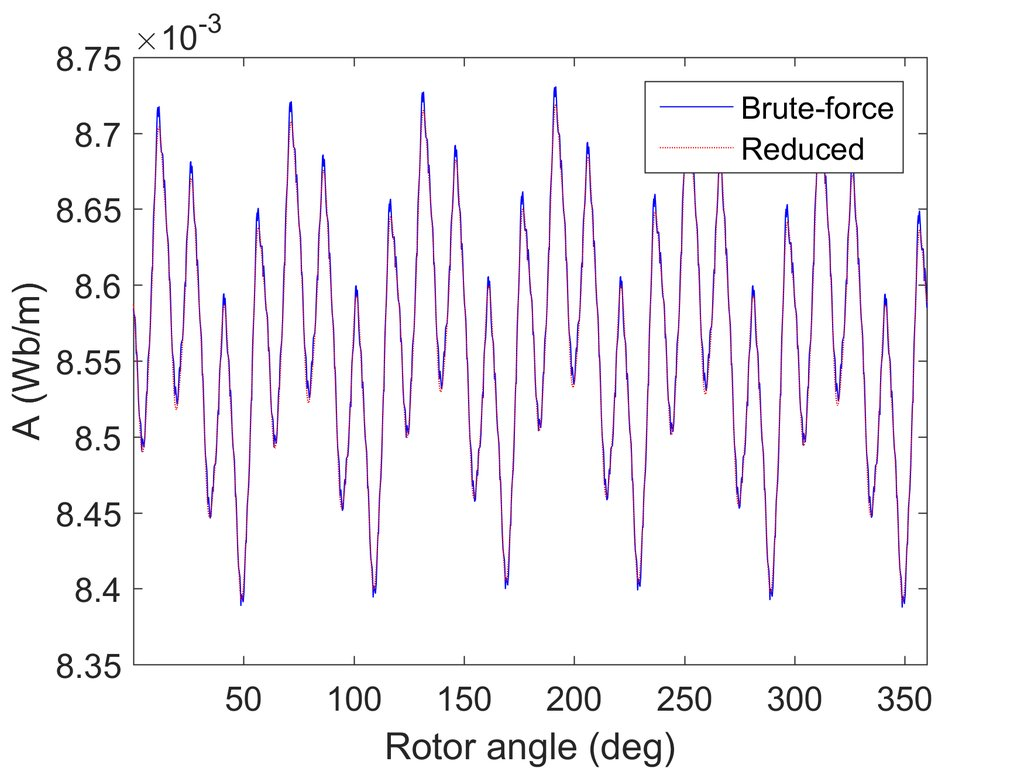Simple time-stepping compared to a reduced-order model.

In the figure, the blue curve has been obtained with the traditional method, with 1000 time-steps. By contrast, the red curve displays the results from a reduced model, roughly corresponding to only 15 steps.

So how was this achieved?

You remember the governing equation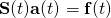for a finite element problem. In the commonly-used time-stepping analysis, the time-varying vector of the nodal potentials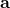is solved again and again for different instants.

However, this approach entails some degree of redundancy. What I mean is that the potentials do not arbitrarily change from one value to another as the timegoes by. Instead, they usually behave in quite a regular fashion.

This is even visible in the figure above. The illustrated potential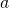(on the rotor surface, remember) is approximately constant with time, only oscillating between 8.4 and 8.7 Wb/m in a very predictable fashion.

More mathematically speaking, we say that the potentialhas only a few significant frequency components. That means it can be written as a Fourier series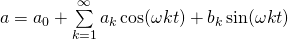with only a few of the coefficients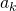,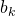significantly different from zero.

Indeed, this fact is often utilized in the harmonic analysis of induction machines, in which the unknown potentials are written with the complex exponential function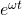as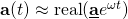,

and the vector of complex coefficients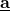is then solved.

And this approach works.

Quite okay.

For induction machines.

The problem is that often the solution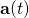can be only poorly approximated by the single-frequency term. For instance, the stator and rotor slots will often create some high-frequency ripple in the solution. Additionally, the magnetic saturation of the iron parts can significantly distort the waveforms, often resulting in a large third harmonic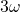in the spectrum.

Perhaps even more importantly, in a synchronous machine the rotor field is almost constant with time (corresponding to a large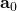term in the Fourier series), whereas the stator field is mostly changing at the fundamental frequency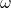. Slotting and saturation also play their roles here, obviously.

Trying to represent the solution with a single-frequency phasorwill fail quite spectacularly in this case. It’s just plain impossible to approximate a signal that has both strong time-varying and time-static components, with another signal that only has one or the other.

Doesn’t work, no matter what you do.

So, today we have concluded that the time-dependent behaviour of an electrical machine could be reasonably approximated as a Fourier series of type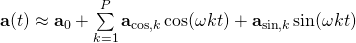,

with only a limited number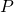of terms. However, we have also seen that for synchronous machines it is absolutely critical to have at least  two frequency components present, namely the DC term and the fundamental. Moreover, some higher harmonics are often also required for a close approximation.

Indeed, in the figure above, that you have seen twice by now, the number of frequency components was 15. But how exactly where the coefficients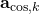,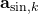calculated?

You’ll see soon enough.

Need help with electric motor design or design software? Let's get in touch - satisfaction guaranteed!Making time-stepping FEM faster, part 2